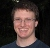# Violation of equality of means in bivariate cholesky model

6 posts / 0 newOffline
Joined: 10/08/2014 - 05:28
Violation of equality of means in bivariate cholesky model

Hi,

I ran the saturated bivariate submodels to check whether the assumptions of equality of means and variance were met, and I noticed something quite interesting: although the submodels were significantly different from the saturated full model when I constrained the means across twins and zygosity, the submodel which constrained means and variances across twins and zygosity was not significantly different from the saturated full model. Are there any possible explanations for this? And can I still go ahead and run the bivariate cholesky models even though the equal means assumption is violated?

Thanks and best regards,
YiOffline
Joined: 03/01/2013 - 11:03
Clarify

Hi Yi,

I want to make sure I understand the question. Say you have these three models

• Sat: full saturated model without constraints
• SatM: saturated model with means equal across twins and zygosity
• SatMV: saturated model with means and variances equal across twins and zygosity

Are you saying you found Sat and SatM were different, but Sat and SatMV were not different?Offline
Joined: 10/08/2014 - 05:28
Yes, that's right

Hi Hunter,

Yes, that's right. Here's the output I got:

> # ------------------------------------------------------------------------------
> # RUN SATURATED SUBMODELS
>
> # Constrain expected Means to be equal across twin order
> eqMeansTwinModel <- mxModel( twinSatModel, name="eqMeTwins" )
> for (i in 1:nv) {
+ eqMeansTwinModel <- omxSetParameters( eqMeansTwinModel, label=c(laMeMZ[nv+i],laMeMZ[i]), free=TRUE, values=svMe[i], newlabels=laMeMZ[i] )
+ eqMeansTwinModel <- omxSetParameters( eqMeansTwinModel, label=c(laMeDZ[nv+i],laMeDZ[i]), free=TRUE, values=svMe[i], newlabels=laMeDZ[i] ) }
> eqMeansTwinFit <- mxRun( eqMeansTwinModel, intervals=T )
Running eqMeTwins

> # Constrain expected Means to be equal across twin order and zygosity
> eqMeansZygModel <- mxModel( eqMeansTwinModel, name="eqMeZyg" )
> for (i in 1:nv) {
+ eqMeansZygModel <- omxSetParameters( eqMeansZygModel, label=c(laMeMZ[i],laMeDZ[i]), free=TRUE, values=svMe[i], newlabels=laMeMZ[i] ) }
> eqMeansZygFit <- mxRun( eqMeansZygModel, intervals=T )
Running eqMeZyg

> # Print Comparative Fit Statistics
> SatNested <- list(eqMeansTwinFit, eqMeansZygFit)
> tableFitStatistics(twinSatFit, SatNested)
Name ep -2LL df AIC diffLL diffdf p
Model 1 : twinSat 28 1104.54 275 554.54 - - -
Model 2 : eqMeTwins 24 1114.98 279 556.98 10.44 4 0.03
Model 3 : eqMeZyg 22 1122.33 281 560.33 17.78 6 0.01

> # Constrain expected Variances to be equal across twin order (and all the means as well..)
> eqVarsTwinModel <- mxModel( eqMeansZygModel, name="eqThTwins" )
> for (i in 1:nv) {
+ #making cov[1,1]=cov[3,3];cov[2,2]=cov[4,4]
+ eqVarsTwinModel <- omxSetParameters( eqVarsTwinModel, label=c(paste("covMZ_",nv+i,"",nv+i,sep=""),paste("covMZ",i,"",i,sep="")),
+ free=TRUE, values=svVa[i], newlabels=paste("covMZ
",i,"",i,sep="") )
+ eqVarsTwinModel <- omxSetParameters( eqVarsTwinModel, label=c(paste("covDZ
",nv+i,"",nv+i,sep=""),paste("covDZ",i,"",i,sep="")),
+ free=TRUE, values=svVa[i], newlabels=paste("covDZ
",i,"_",i,sep="") ) }
> eqVarsTwinFit <- mxRun( eqVarsTwinModel, intervals=T )

Running eqThTwins
> eqVarsTwinSumm <- summary( eqVarsTwinFit )

> tableFitStatistics(twinSatFit, eqVarsTwinFit)
Name ep -2LL df AIC diffLL diffdf p
Model 1 : twinSat 28 1104.54 275 554.54 - - -
Model 2 : eqThTwins 18 1122.49 285 552.49 17.94 10 0.06Offline
Joined: 07/31/2009 - 15:26
Nested vs base comparison

It looks like the eqThTwins-twinSat comparison is just barely not significant. The fit of eqThTwins is essentially the same as eqMeZyg, but eqThTwins uses fewer free parameters. Right now you're comparing everything to the saturated model: a vs b, a vs c, a vs d. If it were me I'd rather compare nested models in sequence: a vs b, b vs c, c vs d. For the results you reported, it would look like this.

Name ep -2LL df AIC diffLL diffdf p
Model 1 : twinSat 28 1104.54 275 554.54 - - -
Model 2 : eqMeTwins 24 1114.98 279 556.98 10.44 4 0.03
Model 3 : eqMeZyg 22 1122.33 281 560.33 7.35 2 0.025
Model 4 : eqThTwins 18 1122.49 285 552.49 0.16 4 0.99

This would suggest you lose important information going from twinSat to eqMeTwins; and going from eqMeTwins to eqMeZyg; but don't lose important information going from eqMeZyg to eqThTwins.Offline
Joined: 10/08/2014 - 05:28
Thank you Michael for your

Thank you Michael for your helpful explanation. Given that I lose important information from twinSat to eqMeTwins, will I still be able to run bivariate ACE model then? Or are there some changes I need to make to the model?Offline
Joined: 02/26/2017 - 16:04